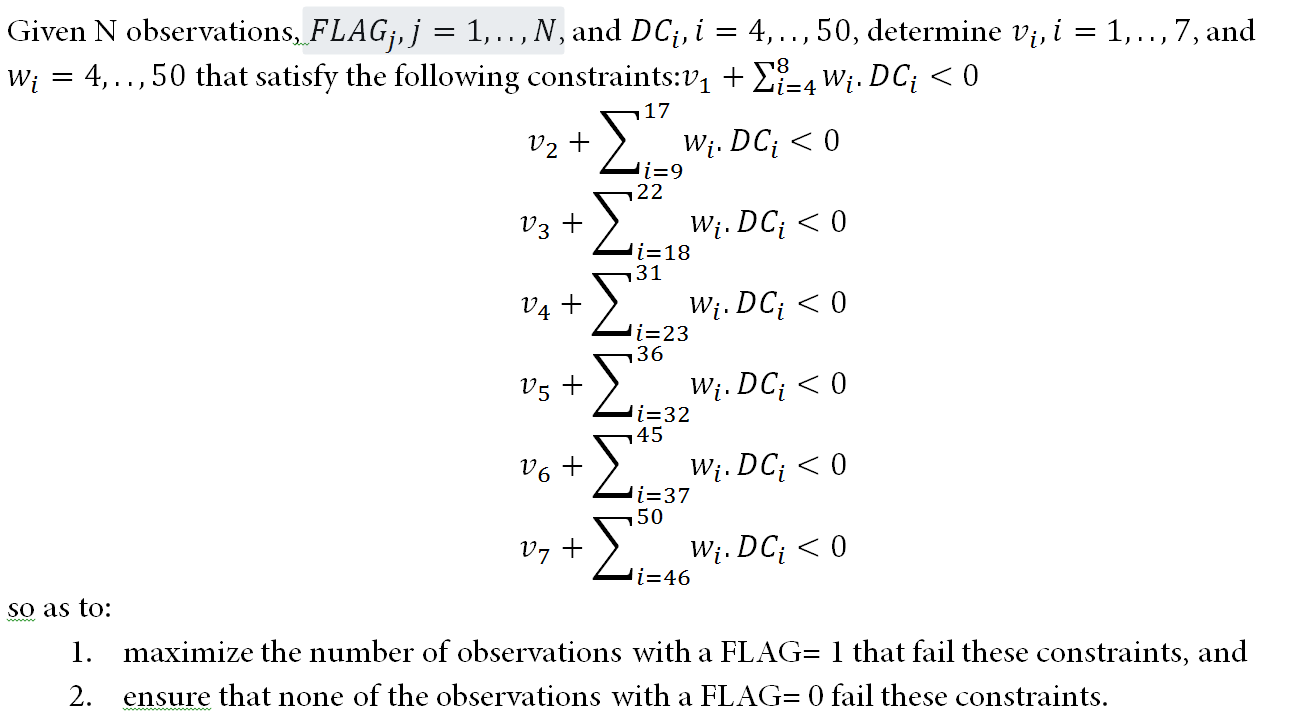## Optimization question

Hello -

I am trying to solve the following problem:I need help identifying the proper procedure and structuring the statements.

Siva

3 REPLIES 3

## Re: Optimization question

You can use PROC OPTMODEL in SAS/OR to formulate the problem and call either the MILP solver or possibly the CLP solver:

SAS/OR(R) 13.2 User's Guide: Mathematical Programming

Besides the v and w decision variables, you need a binary decision variable x[j,k] that indicates whether observation j fails constraint k.  You want to fix x[j,k] = 0 for all observations j with FLAG = 0 and all constraints k.  If you are using the MILP solver, you can enforce this relationship by introducing big-M constraints (assuming DC also depends on j):

/* if x[j,k] = 1 then v + sum {i in ISET} w * DC[i,j] >= 0 */

-v - sum {i in ISET} w * DC[i,j] <= M1[j,k] * (1 - x[j,k])

/* if x[j,k] = 0 then v + sum {i in ISET} w * DC[i,j] < 0 */

v + sum {i in ISET} w * DC[i,j] + epsilon <= M2[j,k] * x[j,k]

where M1[j,k] and M2[j,k] are upper bounds on the left hand sides, and epsilon is a small positive number, say 1e-6, that represents how far away from 0 you consider "negative" to be.

If you are using the CLP solver, you can enforce the relationship with a single set of REIFY constraints, without big-M or epsilon:

REIFY(x[j,k], v + sum {i in ISET} w * DC[i,j] >= 0)

But note that the CLP solver requires all variables to be integer, and I don't know whether your v and w have that restriction.

There is a bit of ambiguity in the problem statement.  Does "fail these constraints" mean fail all constraints or fail at least one constraint?

In either case, you need another binary variable y that indicates whether observation j fails, and your objective is to maximize sum {j in JSET} y.  If y = 1 means fail all, then you want y <= x[j,k] for all k.  If y = 1 means fail at least one, then you want y <= sum {k in KSET} x[j,k].

## Re: Optimization question

RobPratt ..

Regarding the questions in your post..

1. "But note that the CLP solver requires all variables to be integer, and I don't know whether your v and w have that restriction."

In my problem, v and w are real numbers

2. "There is a bit of ambiguity in the problem statement.  Does "fail these constraints" mean fail all constraints or fail at least one constraint?"

You have it right. "Fail these constraints" means "Fail at least one constraint".